## 参数设置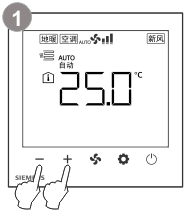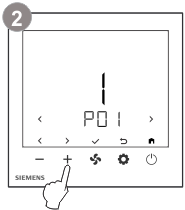或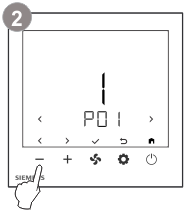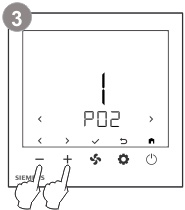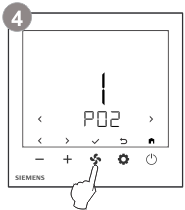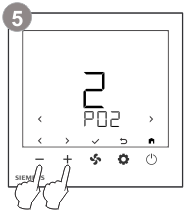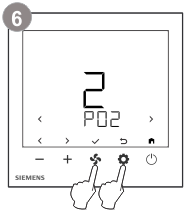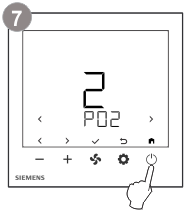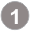同时按下 + 和 - 键 3 秒以上。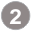初级参数：松开按键且在 2 秒内按下 + 键，直到屏幕显示 Pxx。高级参数：松开按键且在 2 秒内按下 - 键，直到屏幕显示 Pxx。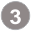按下 + 或 - 键（使用 < 或 > 键）选择参数，所选参数的当前值会显示。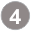按下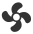键（使用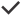键）以编辑所需参数的值。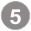在编辑过程中，按下 + 或 - 键增加/减少或选择当前参数的值。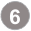按下键（使用键）以确认更改的值，或按下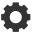键（使用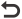键）取消更改。重复步骤 3 到 5，以更改更多参数。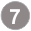按下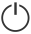键（使用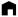键）退出参数设置模式。

## 恢复出厂设置

• 选择参数 P71，并把参数值设置为 1。
• 控制参数的出厂设置将会重新加载。
• 重载过程中，屏幕显示“- - -”。

## 控制参数

P01 *

（设置主/从装置）

1

0 = 自动/制热/制冷/送风/除湿

1 = 制热/制冷/送风/除湿

2 = 制热/制冷/送风

3 = 制热/制冷

4 = 制热/送风

5 = 制冷/送风/除湿

6 = 制冷/送风

P02 *

1

1 = 舒适 – 保护

2 = 舒适 – 节能 – 保护

P03

1

0 = 0.5 K

1 = 1 K

P04

0

0 = ℃（摄氏度）

1 = °F（华氏度）

P05

0

-5…5 K

P06

0

0 = 室内温度

1 = 设定值

2 = 总线温度

P08

21 °C

5…40 ℃

P09

5 °C

5 ℃…P10 (P10 = 40 ℃ 最大值)

P10

35 °C

(P09 = 5 ℃ 最小值) P09…40 ℃

P11

15 °C

5…40 ℃

P14

0

0 = 解锁

1 = 仅调节设定值和风机按键

2 = 全锁

P25 *

0

0 = 禁用

1 = 启用

P26

1

1 = 1:5

2 = 2:4

3 = 3:3

4 = 4:2

5 = 5:1

P27

0

0 = 前一运行模式

1 = 关闭

2 = ON（正常模式）

P28

1

0 = 禁用

1 = 启用

* 这些参数至关重要，必须根据调试期间选择的设备进行设置。

P30

2 K

0.5…6 K

P38 *

0

0 = ---（无功能）

1 = 地暖限定传感器 (NTC 3k)

2 = 地暖限定传感器 (NTC 10k)

P48

1 min

1…20 分钟

P49

1 min

1…20 分钟

P51 *

0

0 = 关闭或 10...50 °C

P53 *

3

1 = 1 速

2 = 2 速

3 = 3 速

4 = 4 速

5 = 5 速

6 = 6 速

7 = 7 速

P54 *

3

1 = 1 速

2 = 2 速

3 = 3 速

4 = DC 0...10 V（ECM 风机）

P55

ECM 风机最大输出

80 %

ECM 风机最小值…100 %

P56

ECM 风机最小输出

30 %

1 %...ECM 风机最大值

P59

2 min

1…6 分钟

P65 *

8 °C

Wcoolprot=40 ℃ 最大

P71

0

0 = 禁用

1 = 开始重载

P81 *

247

1…247

P82 *

Modbus 波特率

2

1 = 9600 bps

2 = 19200 bps

3 = 38400 bps

4 = 57600 bps

P83 *

Modbus 数据帧格式

0

0 = 1/8/E/1

1 = 1/8/O/1

2 = 1/8/N/1

3 = 1/8/N/2

P98 *

1

1 = 启用

* 这些参数至关重要，必须根据调试期间选择的设备进行设置。

d02

X1 状态

d06

d07

0 = 0 % 输出

1...100 = 1 % 至 100 % 输出

P53

d08

Q1 至 Q3 是常开继电器

0 = 所有继电器打开

1 = 仅闭合 Q1

2 = 仅闭合 Q2

3 = 仅闭合 Q3

5 = 仅闭合 Q5

6 = 所有继电器闭合

P25, P54

d10

FAU – 激活 EXT/INT 循环风阀

OPE = 开启（正常位置）

CLO = 关闭

P25

d12

OPE = 开启

CLO = 关闭